Find Paper, Faster
Example：10.1021/acsami.1c06204 or Chem. Rev., 2007, 107, 2411-2502
CHAIN COMPONENTS WITH THE STABLE SHADOWING PROPERTY FOR C1 VECTOR FIELDS
Journal of the Australian Mathematical Society  (IF0.8),  Pub Date : 2021-02-01, DOI: 10.1017/s1446788720000415
MANSEOB LEE, LE HUY TIEN

Let M be a closed n-dimensional smooth Riemannian manifold, and let X be a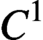$C^1$-vector field of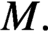$M.$ Let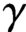$\gamma$ be a hyperbolic closed orbit of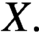$X.$ In this paper, we show that X has the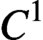$C^1$-stably shadowing property on the chain component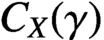$C_X(\gamma )$ if and only if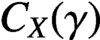$C_X(\gamma )$ is the hyperbolic homoclinic class.